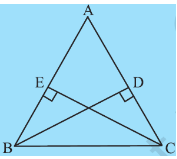# 3. In Fig 7.33, and are altitudes of  such that .          (i) State the three pairs of equal parts in and .           (ii) Is  ? Why or why not?           (iii) Is  ? Why or why not?P Pankaj Sanodiya

i) Given,  in and .

ii) So, By RHS Rule of congruency, we conclude:

iii) Since both the triangle are congruent, all parts of one triangle are equal to their corresponding part from another triangle.

So.

.

Exams
Articles
Questions